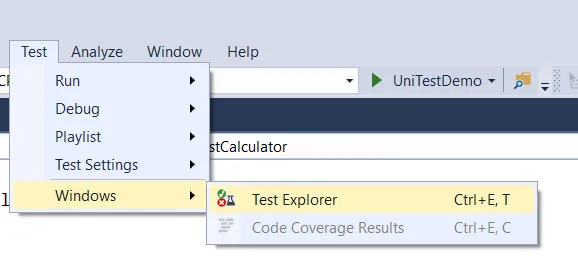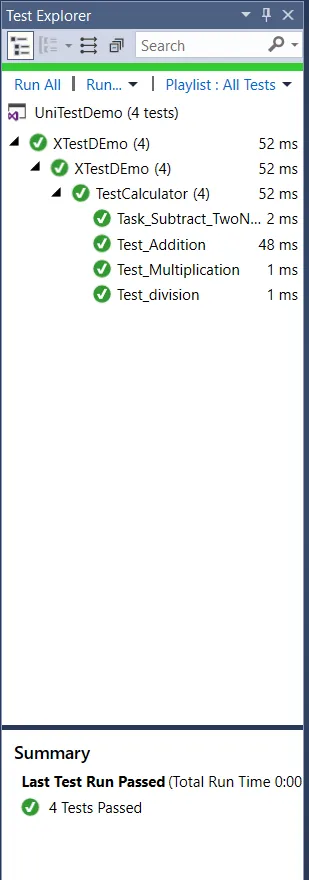﻿ Integration of unit testing with ASP.NET Core

# Integration of unit testing with ASP.NET Core

## Straighten your back and cherish with coffee - PLAY !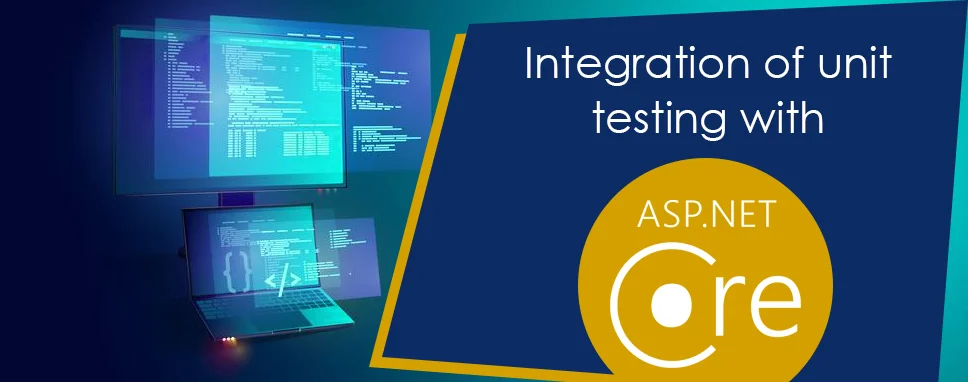Unit testing is used to test individual components or units to determine if there are any issues by the developer himself. The unit testing is used to validate that each component of the software code performs as expected. The unit testing is concerned with the functional correctness of standalone modules and it is done by the developer during the development phase of an application.

## Why do we need unit tests?

Unit testing is important because the software can fail in unexpected ways. Manual testing is slow, least reliable, and expensive to test an application. Whereas, unit testing saves time and money if it is implemented properly.

Here, are a few key reasons to perform unit testing:

1. Unit tests are used to fix bugs early in the development phase and save costs and time.
2. It is used by the developers to understand the code base and then it enables them to make changes quickly.
3. Good unit testing serves as project documentation of software.
4. Unit tests are used to re-use code and they are easy as well to identify defects in any application.

## Unit Testing Tools

There are many automated tools available to test the application.

Few examples as below:

#### 1) JunitJunit is a free testing tool used by the developer for Java programming language. It implements assertions to identify the test method. Junit is used to test data first and then inserted in the piece of code.

#### 2) NUnitNUnit is a framework widely used by the developer to unit-test an application that is developed in all .NET languages. Nunit is open source and supports data-driven tests that run parallelly. Nunit allows the developer to write a script manually.

#### 3) PHPUnitPHPUnit is a unit testing tool used by the developer to test an application. PHPUnit tests a small portion of code known as units. PHPUnit provides a pre-define assertion to assert that the system behaves in a proper manner.

#### 4) JMockitJMockit is open-source Unit testing with line and path metrics. JMockit allows the developer to record and verifying syntax in API and offers Line coverage, Path Coverage, and Data Coverage.

#### 5) EMMAEMMA is an open-source toolkit used by the developer for analyzing and reporting code written in Java language. Emma is a java-based external library and support method, line, basic block coverage type.

## Setting up Unit testing in ASP.NET Core Application

There are many test frameworks available in the market but we will be going to use the xUnit, which is a very popular testing framework. The simplest way to create unit test case for an ASP.NET Core web app project is to create a new test project using a template.

We can create a test class for each application class. The simple unit test includes three-step:

1. Arrange:In this step setup the necessary variable and object.
2. Act:In this step, call the method with a required parameter for testing
3. Assert:In this step, the expected result is verified

## Create a sample ASP.NET Core application

To create ASP.NET Core Console Application follow the below steps:

Step 1: Open Visual Studio, select File option -> New option -> Project

Step 2:Select the ASP.NET Core Console Application template and click Next.

Step 3:Enter the project name, and then click Create.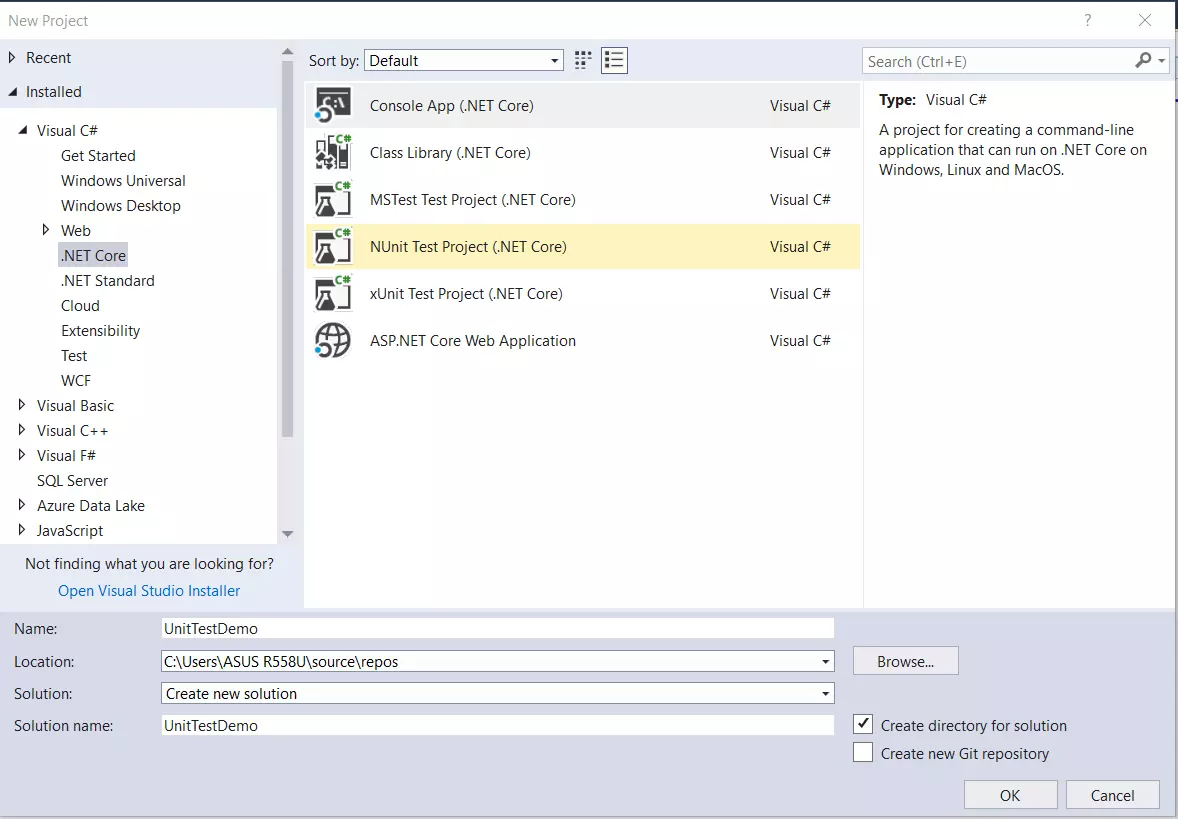Figure 1 Create an application

Step 4: Create a new class

```namespace UniTestDemo
{
public static class Calculator
{
public static double Addition(double num1, double num2)
{
return (num1 + num2);
}

public static double Subtraction(double num1, double num2)
{
return (num1 - num2);
}

public static double Multiplication(double num1, double num2)
{
return (num1 * num2);
}

public static double Division(double num1, double num2)
{
return (num1 / num2);
}
}
}
```

1: In Visual Studio 2019, Right-click on solution-> Add -> Project.

2: In the Create New Project dialog, select the Test ->xUnit Test Project (.Net Core) template, and click Next.

3: Enter the project name, and then click Create.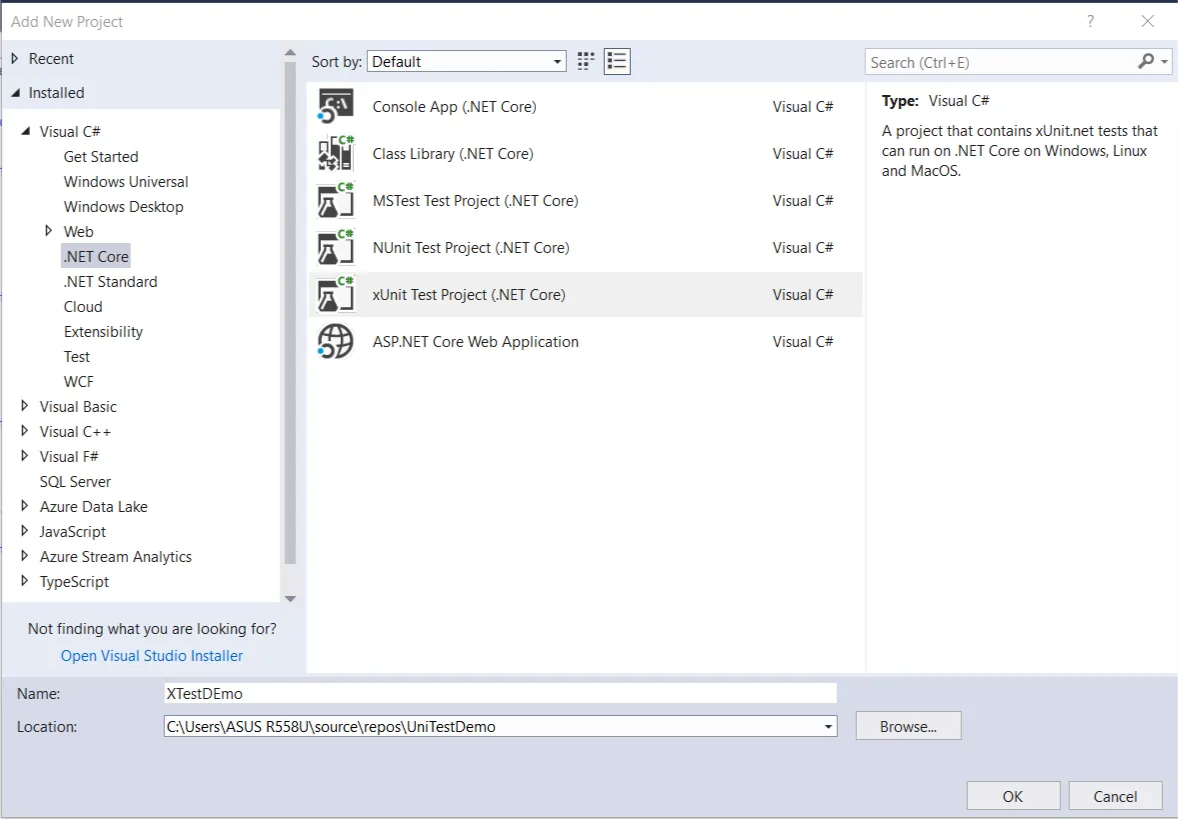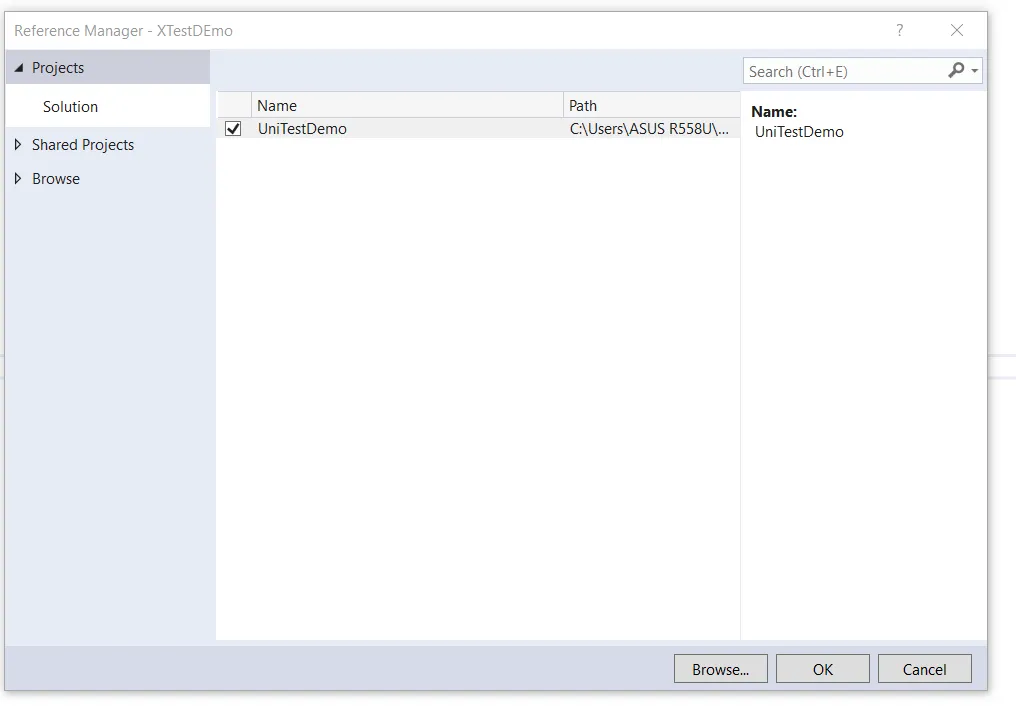Figure 3 Add Reference to the testing project

## Searching for Dedicated ASP.Net Core Web Developer ?

Step 6: Now, Add one class in 'XTestDEmo' project named 'TestCalculator.cs'

In 'TestCalculator.cs' class we will write test case for method define in 'Calculator.cs'. These methods follow three-step: Arrange, Act, Assert.

The following class contains test cases for Calculator:

```using System;
using UniTestDemo;
using Xunit;

namespace XTestDEmo
{
public class TestCalculator
{
[Fact]
{
// Arrange
var number1 = 4.9;
var number2 = 3.1;
var expectedValue = 8;

// Act

//Assert
Assert.Equal(expectedValue, sum, 1);
}

[Fact]
{
// Arrange
var number1 = 3.2;
var number2 = 1.5;
var expectedValue = 1.7;

// Act
var sub = Calculator.Subtraction(number1, number2);

//Assert
Assert.Equal(expectedValue, sub, 1);
}

[Fact]
public void Test_Multiplication()
{
// Arrange
var number1 = 2.5;
var number2 = 3.5;
var expectedValue = 8.75;

// Act
var mult = Calculator.Multiplication(number1, number2);

//Assert
Assert.Equal(expectedValue, mult, 2);
}

[Fact]
public void Test_division()
{
// Arrange
var number1 = 2.9;
var number2 = 3.1;
var expectedValue = 0.94; //Rounded value

// Act
var div = Calculator.Division(number1, number2);

//Assert
Assert.Equal(expectedValue, div, 2);
}
}
}
```

Step 7: Now, Run your test cases

For running all test cases open Test Explorer where you will find you're all test case. To run click on RunAll this will start executing test cases.

To open Test Explorer in visual studio Click on Test option->Window->Test Explorer.